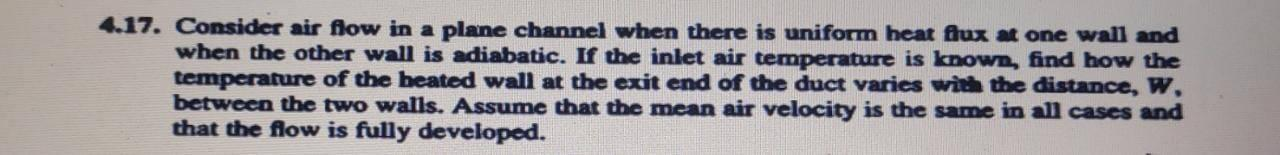# Question Solved1 AnswerConvective Heat transfer 4.17. Consider air flow in a plane channel when there is uniform heat flux at one wall and when the other wall is adiabatic. If the inlet air temperature is known, find how the temperature of the heated wall at the exit end of the duct varies with the distance, w between the two walls. Assume that the mean air velocity is the same in all cases and that the flow is fully developed.HGJ3KN The Asker · Mechanical Engineering

Convective Heat transferTranscribed Image Text: 4.17. Consider air flow in a plane channel when there is uniform heat flux at one wall and when the other wall is adiabatic. If the inlet air temperature is known, find how the temperature of the heated wall at the exit end of the duct varies with the distance, w between the two walls. Assume that the mean air velocity is the same in all cases and that the flow is fully developed.
More
Transcribed Image Text: 4.17. Consider air flow in a plane channel when there is uniform heat flux at one wall and when the other wall is adiabatic. If the inlet air temperature is known, find how the temperature of the heated wall at the exit end of the duct varies with the distance, w between the two walls. Assume that the mean air velocity is the same in all cases and that the flow is fully developed.